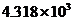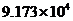Chapter 10, Problem 30QAP### Introductory Chemistry: A Foundati...

9th Edition
Steven S. Zumdahl + 1 other
ISBN: 9781337399425

#### Solutions

Chapter
Section### Introductory Chemistry: A Foundati...

9th Edition
Steven S. Zumdahl + 1 other
ISBN: 9781337399425
Textbook Problem
1 views

# Perform the indicated conversions. 91.74 kcal into calories 1.781 U into calories i>J into kilocaloriesi>cal into kilojoules

Interpretation Introduction

(a)

Interpretation:

Convert 91.74 kilocalories to calories.

Concept Introduction:

A conversion factor is a numerical ratio which is used to show a measurement in one unit as another unit. It is always equal to 1.

Explanation

The given conversion is: conversion of kilocalories to calories.

1 kilocalorie is equal to 1000 calories.

1 kcal = 1000 cal

Thus, conversion factor is given by:

1000 cal1 kcal

So,

91

Interpretation Introduction

(b)

Interpretation:

Convert 1.781 kilojoules to calories.

Concept Introduction:

A conversion factor is a numerical ratio which is used to show a measurement in one unit as another unit. It is always equal to 1.

Interpretation Introduction

(c)

Interpretation:

Convert 4.318×103J to kilocalories.

Concept Introduction:

A conversion factor is a numerical ratio which is used to show a measurement in one unit as another unit. It is always equal to 1.

Interpretation Introduction

(c)

Interpretation:

Convert 9.173×104 cal to kilojoules.

Concept Introduction:

A conversion factor is a numerical ratio which is used to show a measurement in one unit as another unit. It is always equal to 1.

### Still sussing out bartleby?

Check out a sample textbook solution.

See a sample solution

#### The Solution to Your Study Problems

Bartleby provides explanations to thousands of textbook problems written by our experts, many with advanced degrees!

Get Started

#### Find more solutions based on key concepts